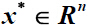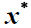# 随机对称不确定集下的线性互补问题Linear Complementarity Problems under a Random Symmetric Uncertainty

DOI: 10.12677/AAM.2016.51001, PDF, HTML, XML, 下载: 1,746  浏览: 3,835  国家自然科学基金支持

Abstract: In this paper, we introduce the notion of robust solution of uncertain linear complementarity problems. We prove that, if robust counterpart to uncertain quadratic programming—a robust optimization problem, has a optimal solution, and the optimum value equals to zero, thenis the robust solution of the uncertain linear complementarity problem. By probability theory, we discuss linear complementarity problems under a random symmetric uncertainty, and obtain sufficient and necessary conditions of almost reliable robust solution.

  Ben-Tal. A. and Nemirovski. A. (1997) Stable Truss Topology Design via Semidefinite Programming. SIAM Journal on Optimization, 7, 991-1016. http://dx.doi.org/10.1137/S1052623495291951  Ben-Tal, A. and Nemirovski, A. (1998) Robust Convex Optimization. Mathematics of Operations Research, 23, 769- 805. http://dx.doi.org/10.1287/moor.23.4.769  Ben-Tal, A. and Nemirovski, A. (2000) Robust Solutions of Linear Programming Problems Contaminated with Uncertain Data. Mathematical Programming, 88, 411-424. http://dx.doi.org/10.1007/PL00011380  Ben-Tal, A., El-Ghaoui, L. and Nemirovski, A. (2000) Robust Semi-definite Programming. Kluwer Dordrecht.  Fang, H., Chen, X. and Fukushima, M. (2007) Stochastic Matrix Linear Complementarity Problems. SIAM Journal on Optimization, 18, 482-506. http://dx.doi.org/10.1137/050630805  Ferris, M.C. and Pang, J.S. (2010) Engineering and Economic Appli-cations of Complementarity Problems. SIAM Review, 39-69.  Goldfarb, D. and Iyengar, G. (2010) Robust Portfolio Selection Problems. Mathematics of Operations Research, 28, 1-37. http://dx.doi.org/10.1287/moor.28.1.1.14260